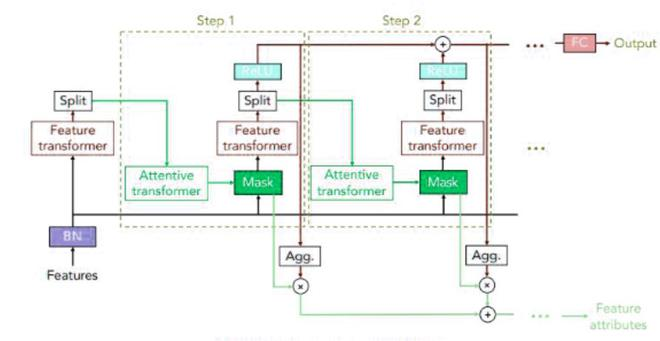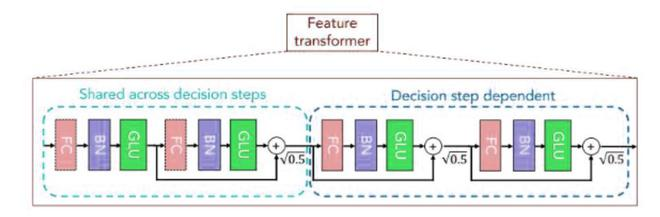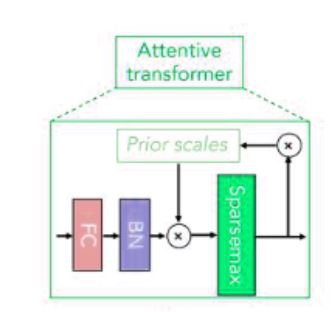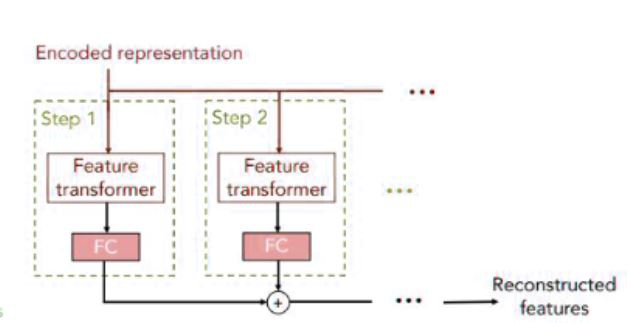# TabNet

• Last Updated : 16 Oct, 2021

TabNet was proposed by the researchers at Google Cloud in the year 2019. The idea behind TabNet is to effectively apply deep neural networks on tabular data which still consists of a large portion of users and processed data across various applications such as healthcare, banking, retail, finance, marketing, etc.

One motivation to apply deep learning to the tabular dataset comes from other domains such as (image, language, speech) data when applied on it demonstrated a significant performance improvement on the large datasets as compared to other machine learning techniques. So, we can expect it to work on tabular data. Another reason can be those tree-based algorithms unlike the deep neural network do not efficiently learn to reduce the error by using techniques like Gradient Descent.

TabNet provides a high-performance and interpretable tabular data deep learning architecture. It uses a method called sequential attention mechanism to enabling which feature to choose to cause high interpretability and efficient training.

### Architecture:

#### TabNet EncoderTabNet Encoder Architecture

The TabNet architecture basically consists of multi-steps which are sequential, passing the input from one step to another. There are different ways to decide the number of steps depending upon the capacity. Each step consists of the following steps:

• In the initial step, the complete dataset is input into the model without any feature engineering. It is then passed through a batch normalization layer, and after that, it is then passed in a feature transformer.
• Feature Transformer:  It consists of n-number (eg. 4) of different GLU blocks. Each GLU bloc consists  of the following layers:
GLU block =  Fully-Connected -  Batch Normalization - GLU (Gated Linear Unit)

where , GLU(x)  =For 4 layers of GLU blocks, the 2 GLU blocks should be shared and 2 should be independent, which helps in robust and efficient learning. There is a skip connection also existed b/w two consecutive blocks. After each block, we perform the normalization within order to get the stability and ensuring that the variance doesn’t vary considerably. The feature transformer outputs the two outputs:

1.It is the output decision from the particular step giving its prediction of continuous values/ classes.
2.The output for the next attentive transformer where the next cycle begins.Feature Transformer

• Attentive Transformer:  An attentive transformer consists of a fully connected (FC) layer, a BatchNorm layer, and Prior scales layer, and a Sparsemax layer. It receives input \mathbb{n_a} and after passing through the fully connected layer and Batch normalization layer, then it passes through the prior scales layer.
• This prior scale layer aggregates how much each feature has been used before the current decision step.;  all features are equal.

P_i = \prod_{j=1}^{i} (\gamma – M ) ; the smaller the value of \gamma the more independent step,looking at different features.

Sparsemax layer: It is used for normalization of the coefficient (similar to softmax), resulting in sparse selection of features:If a lot of the features will be zeros, then we will apply instance-wise feature selection, where only a subset of different features is used for different steps.Attentive transformer

• Attention Mask: The output from the attentive transformer step, are then fed into the a attention mask, which it helps in identify the selected features. It quantifies aggregate feature importance in addition to analysis of each step. Combining the masks at different steps requires a coefficient that can weigh the relative importance of each step in the decision. Therefore, the author proposes:denote the aggregate decision contribution at ith decision step for the bth sample. Scaling the decision mask at each decision step with, the author propose the aggregate feature important mask:#### TabNet DecoderTabNet Decoder

The TabNet decoder architecture consists of a feature transformer, followed by the fully connected layers at the decision step. The output is then summed to the reconstructed features. The reconstruction loss function in self-supervised phase:### Implementation

We will be using the Pytorch implementation of the TabNet in this implementation. For datasets, we will be using the Loan Approval prediction, whether a person will get a loan or not which it applied for:

## Python3

 # Install TabNetpip install pytorch-tabnet  # imports necessary modulesfrom pytorch_tabnet.tab_model import TabNetClassifier  import osimport torchimport pandas as pdimport numpy as np  from sklearn.model_selection import KFoldfrom sklearn.preprocessing import LabelEncoder, MinMaxScalarfrom sklearn.metrics import accuracy_score  # Load training and test datadata =pd.read_csv('/content/train.csv')data.head()data.isna().sum()# load test datatest_data = pd.read_csv('/content/test.csv')test_data.head()test_data.isna().sum()  # set index columndata.set_index('Loan_ID', inplace=True)test_data.set_index('Loan_ID', inplace=True)  # Replace NAsdata.fillna(method="bfill", inplace=True)test_data.fillna(method="bfill", inplace=True)  # convert categorical column to integer Labels gen = LabelEncoder().fit(data['Gender'])data['Gender'] = gen.transform(data['Gender'])  s_type= LabelEncoder().fit(data['Married'])data['Married'] = s_type.transform(data['Married'])  n_dep= LabelEncoder().fit(data['Dependents'])data['Dependents'] = n_dep.transform(data['Dependents'])  edu= LabelEncoder().fit(data['Education'])data['Education'] = edu.transform(data['Education'])  s_emp = LabelEncoder().fit(data['Self_Employed'])data['Self_Employed'] = s_emp.transform(data['Self_Employed'])  c_history = LabelEncoder().fit(data['Credit_History'])data['Credit_History'] = c_history.transform(data['Credit_History'])  p_area = LabelEncoder().fit(data['Property_Area'])data['Property_Area'] = p_area.transform(data['Property_Area'])  l_status = LabelEncoder().fit(data['Loan_Status'])data['Loan_Status'] = l_status.transform(data['Loan_Status'])  # For test datatest_data['Gender'] = gen.transform(test_data['Gender'])test_data['Married'] = s_type.transform(test_data['Married'])test_data['Dependents'] = n_dep.transform(test_data['Dependents'])test_data['Education'] = edu.transform(test_data['Education'])test_data['Self_Employed'] = s_emp.transform(test_data['Self_Employed'])test_data['Credit_History'] = c_history.transform(test_data['Credit_History'])test_data['Property_Area'] = p_area.transform(test_data['Property_Area'])  # select feature and target variableX = data.loc[:,data.columns != 'Loan_Status']y = data.loc[:,data.columns == 'Loan_Status']X.shape, y.shape  # convert to numpyX= X.to_numpy()y= y.to_numpy()  y= y.flatten()  # define and train the Tabnet model with cross validationkf = KFold(n_splits=5, random_state=42, shuffle=True)CV_score_array    =[]for train_index, test_index in kf.split(X):    X_train, X_valid = X[train_index], X[test_index]    y_train, y_valid = y[train_index], y[test_index]    tb_cls = TabNetClassifier(optimizer_fn=torch.optim.Adam,                       optimizer_params=dict(lr=1e-3),                       scheduler_params={"step_size":10, "gamma":0.9},                       scheduler_fn=torch.optim.lr_scheduler.StepLR,                       mask_type='entmax' # "sparsemax"                       )    tb_cls.fit(X_train,y_train,               eval_set=[(X_train, y_train), (X_val, y_val)],               eval_name=['train', 'valid'],               eval_metric=['accuracy'],               max_epochs=1000 , patience=100,               batch_size=28, drop_last=False)                CV_score_array.append(tb_cls.best_cost)  # Test model and generate predictionpredictions =[ 'N' if i < 0.5 else 'Y' for i in tb_cls.predict(X_test)]
Collecting pytorch-tabnet
Requirement already satisfied: numpy<2.0,>=1.17 in /usr/local/lib/python3.7/dist-packages (from pytorch-tabnet) (1.19.5)
Requirement already satisfied: scikit_learn>0.21 in /usr/local/lib/python3.7/dist-packages (from pytorch-tabnet) (0.22.2.post1)
Requirement already satisfied: torch<2.0,>=1.2 in /usr/local/lib/python3.7/dist-packages (from pytorch-tabnet) (1.9.0+cu102)
Requirement already satisfied: scipy>1.4 in /usr/local/lib/python3.7/dist-packages (from pytorch-tabnet) (1.4.1)
Requirement already satisfied: tqdm<5.0,>=4.36 in /usr/local/lib/python3.7/dist-packages (from pytorch-tabnet) (4.62.2)
Requirement already satisfied: joblib>=0.11 in /usr/local/lib/python3.7/dist-packages (from scikit_learn>0.21->pytorch-tabnet) (1.0.1)
Requirement already satisfied: typing-extensions in /usr/local/lib/python3.7/dist-packages (from torch<2.0,>=1.2->pytorch-tabnet) (3.7.4.3)
Installing collected packages: pytorch-tabnet
Successfully installed pytorch-tabnet-3.1.1
# train data
Loan_ID    Gender    Married    Dependents    Education    Self_Employed    ApplicantIncome    CoapplicantIncome    LoanAmount    Loan_Amount_Term    Credit_History    Property_Area    Loan_Status
0    LP001002    Male    No    0    Graduate    No    5849    0.0    NaN    360.0    1.0    Urban    Y
1    LP001003    Male    Yes    1    Graduate    No    4583    1508.0    128.0    360.0    1.0    Rural    N
2    LP001005    Male    Yes    0    Graduate    Yes    3000    0.0    66.0    360.0    1.0    Urban    Y
3    LP001006    Male    Yes    0    Not Graduate    No    2583    2358.0    120.0    360.0    1.0    Urban    Y
4    LP001008    Male    No    0    Graduate    No    6000    0.0    141.0    360.0    1.0    Urban    Y

# null values
Loan_ID               0
Gender               13
Married               3
Dependents           15
Education             0
Self_Employed        32
ApplicantIncome       0
CoapplicantIncome     0
LoanAmount           22
Loan_Amount_Term     14
Credit_History       50
Property_Area         0
Loan_Status           0
dtype: int64
# test data
Loan_ID    Gender    Married    Dependents    Education    Self_Employed    ApplicantIncome    CoapplicantIncome    LoanAmount    Loan_Amount_Term    Credit_History    Property_Area
0    LP001015    Male    Yes    0    Graduate    No    5720    0    110.0    360.0    1.0    Urban
1    LP001022    Male    Yes    1    Graduate    No    3076    1500    126.0    360.0    1.0    Urban
2    LP001031    Male    Yes    2    Graduate    No    5000    1800    208.0    360.0    1.0    Urban
3    LP001035    Male    Yes    2    Graduate    No    2340    2546    100.0    360.0    NaN    Urban
4    LP001051    Male    No    0    Not Graduate    No    3276    0    78.0    360.0    1.0    Urban

# Null values
Loan_ID               0
Gender               11
Married               0
Dependents           10
Education             0
Self_Employed        23
ApplicantIncome       0
CoapplicantIncome     0
LoanAmount            5
Loan_Amount_Term      6
Credit_History       29
Property_Area         0
dtype: int64
# x, y shape
((614, 11), (614, 1))
Device used : cpu
Early stopping occurred at epoch 137 with best_epoch = 37 and best_valid_accuracy = 0.84416
Best weights from best epoch are automatically used!
Device used : cpu
Early stopping occurred at epoch 292 with best_epoch = 192 and best_valid_accuracy = 0.86364
Best weights from best epoch are automatically used!
Device used : cpu
Early stopping occurred at epoch 324 with best_epoch = 224 and best_valid_accuracy = 0.85065
Best weights from best epoch are automatically used!
Device used : cpu
Early stopping occurred at epoch 143 with best_epoch = 43 and best_valid_accuracy = 0.84416
Best weights from best epoch are automatically used!
Device used : cpu
Early stopping occurred at epoch 253 with best_epoch = 153 and best_valid_accuracy = 0.84416
Best weights from best epoch are automatically used!

## References:

My Personal Notes arrow_drop_up